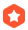# 如何算不规则多边形的面积？cabbagebai 2001-07-29 02:23:33加精
as title
...全文
151 点赞 收藏 7

7 条回复

starfish 2001-07-29
/******************************************** * *
* 计算多边形的面积 *
* *
* 要求按照逆时针方向输入多边形顶点 *
* 可以是凸多边形或凹多边形 *
* *
\********************************************/

float area_of_polygon(int vcount,float x[],float y[])
{
int i;
float s;
if (vcount<3) return 0;
s=y*(x[vcount-1]-x);
for (i=1;i<vcount;i++)
s+=y[i]*(x[(i-1)]-x[(i+1)%vcount]);
return s/2;
}

Arter 2001-07-29
1.把（xi,yi)按逆时针排好。(i=1,2,3,....n, x(n+1)=x1);
2.计算它的有向面积:
(1)先看:(0,0),(xj,yj),(xk,yk)三点的面积S=
¦ 0 0 1 ¦
¦ xj yj 1 ¦*(1/2) =(xj*yk-yj*xk)/2
¦ xk yk 1 ¦
(2)多边形面积可以划分为三角形面积的和:
S[（0，0），（x1,y1),(x2,y2)]+S[（0，0），（x2,y2),(x3,y3)]+...
+ S[（0，0），（x(n-1),y(n-1),(xn,yn)]+S[（0，0），（xn,yn),(x1,y1)]
=((x1*y2-x2*y1)+(x2*y3-x3*y2)+...+(x(n-1)*yn-xn*y(n-1))+(xn*y1-x1*yn))/2
=(x1*(y2-yn)+x2*(y3-y1)+x3*(y4-y2)+...+x(n-1)*（yn-y(n-2))+xn*(y1-y(n-1)))/2
（或：(y1*(xn-x2)+y2*(x1-x3)+y3*(x2-x4)+...+y(n-1)*(x(n-2)-xn)+yn*(x(n-1)-
x1))/2 )

along_zju 2001-07-29
1/2*|( |x0 y0| + |x1 y1| + .... + |xn yn| )|
| |x1 y1| |x2 y2| |x0 y0| |

3.2w+